# Econometrics ch4

8 Sep 2016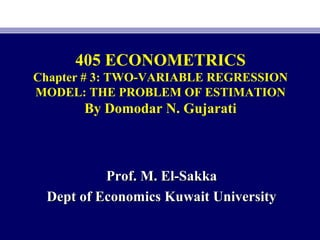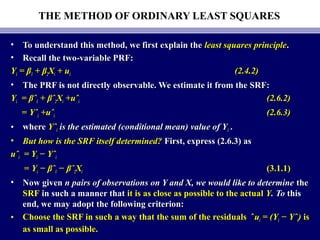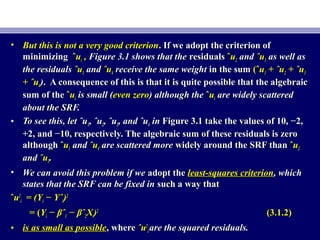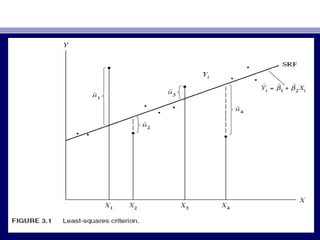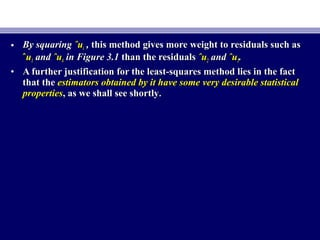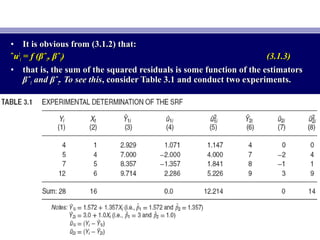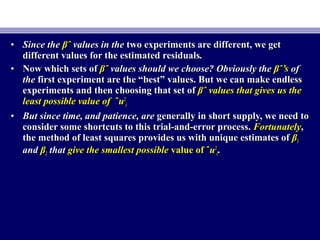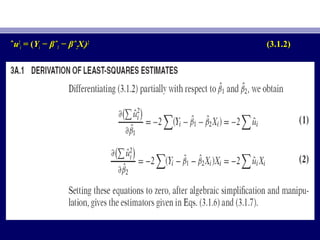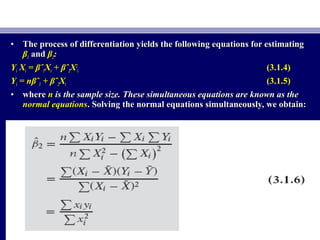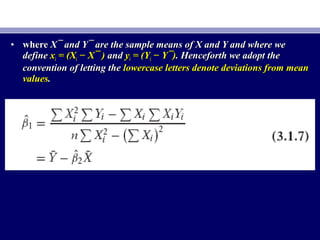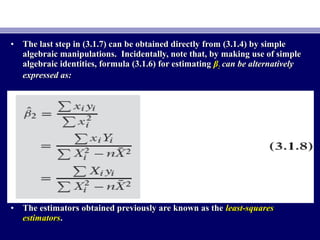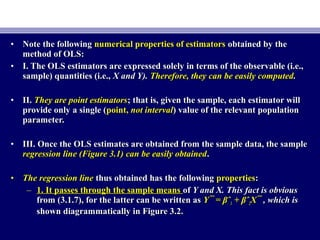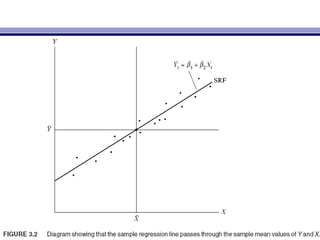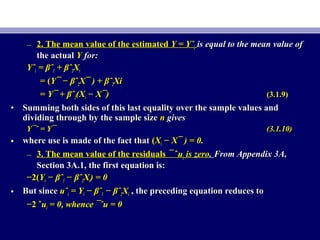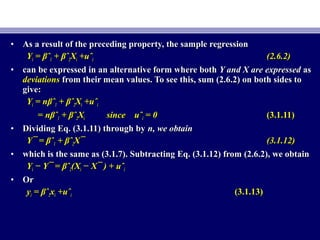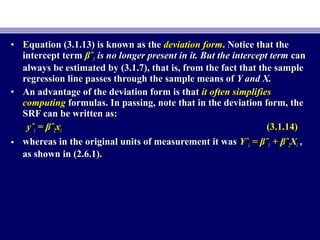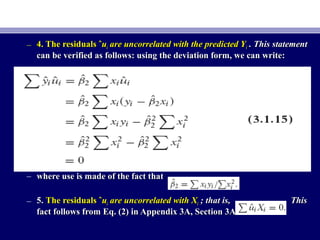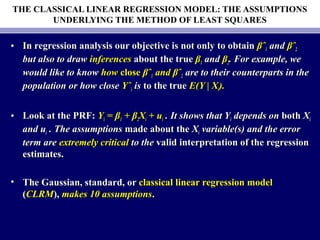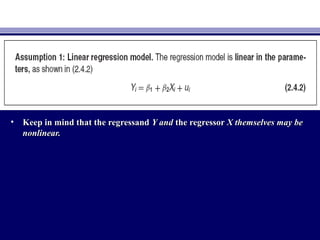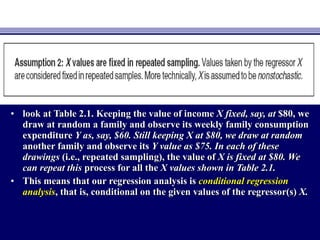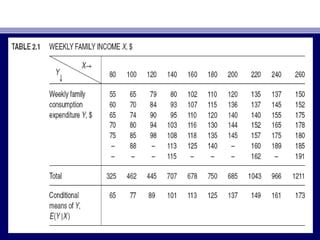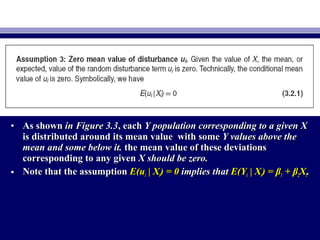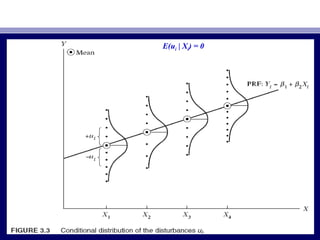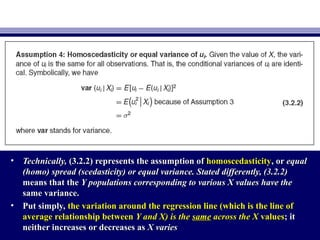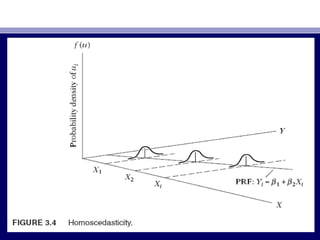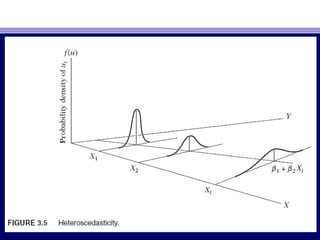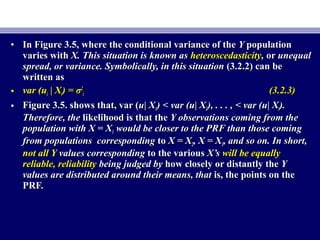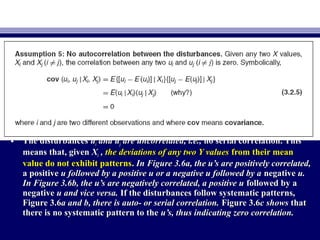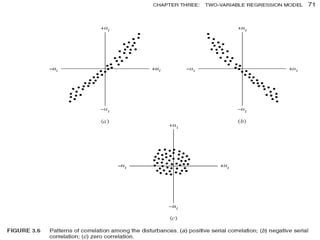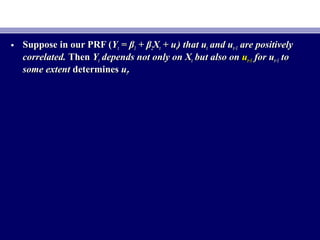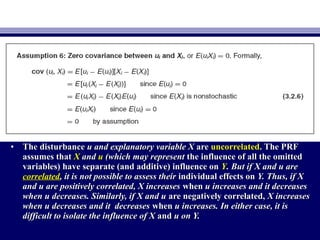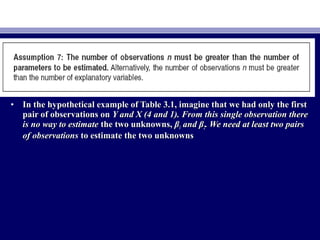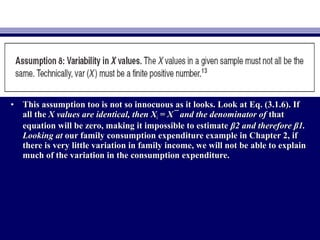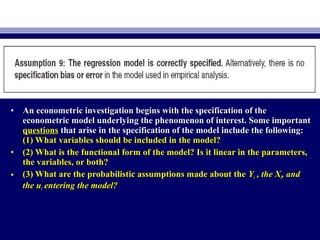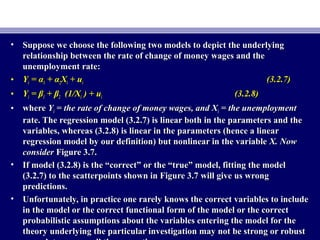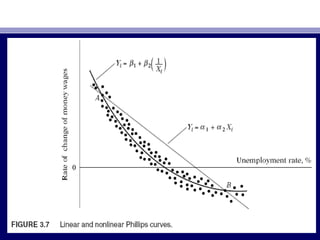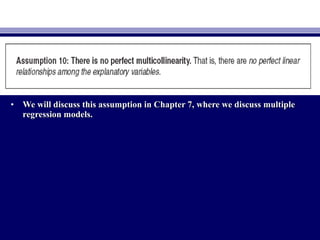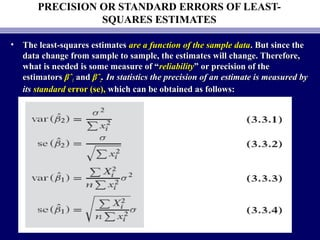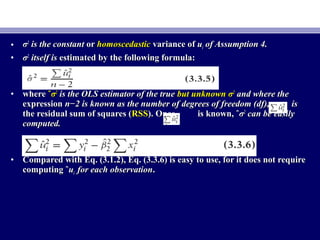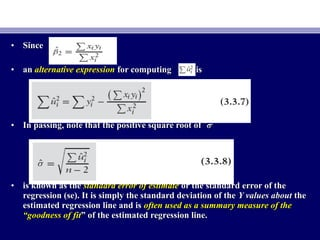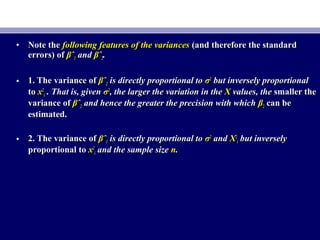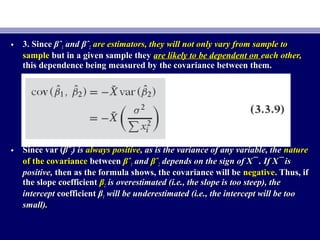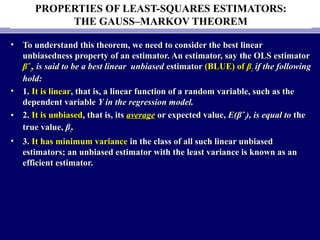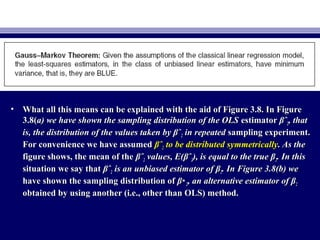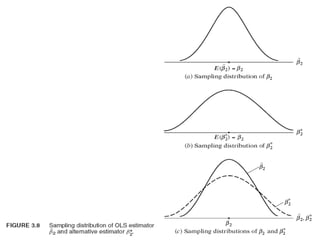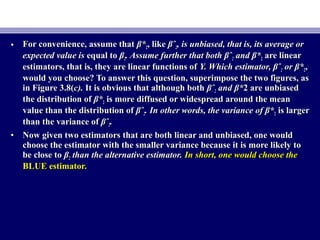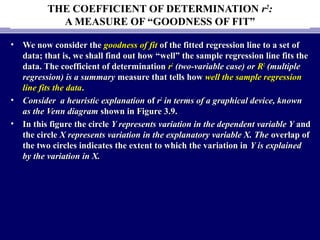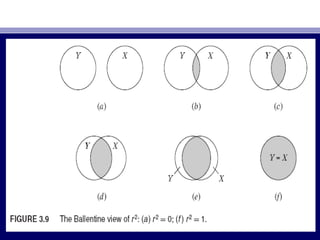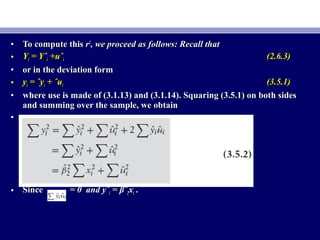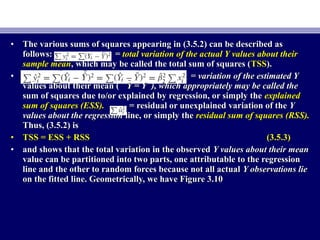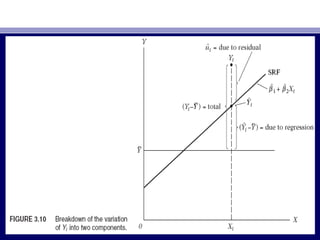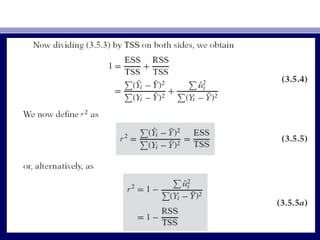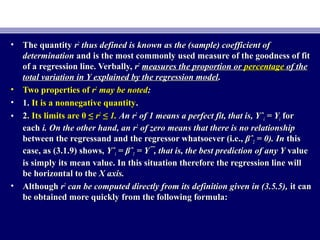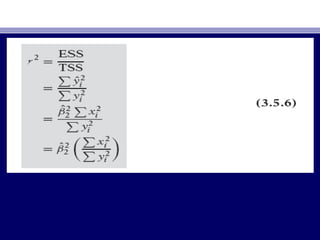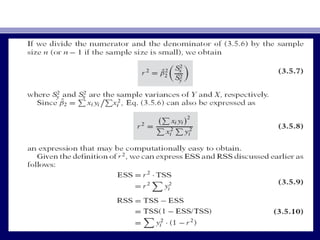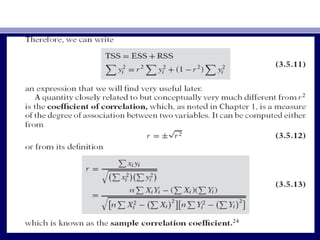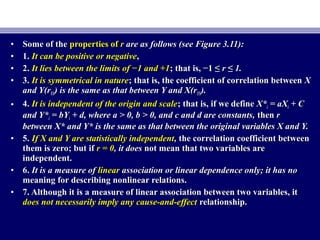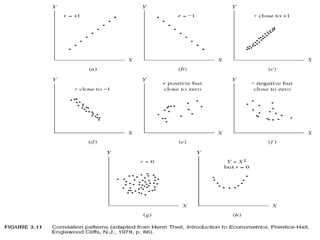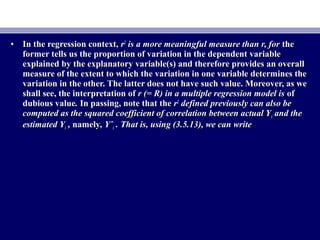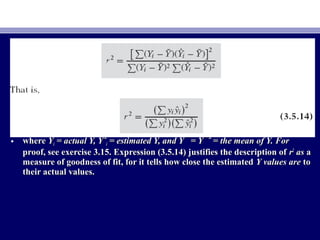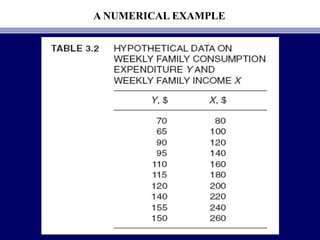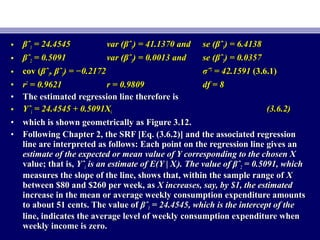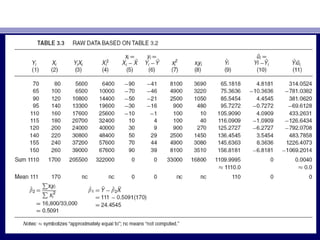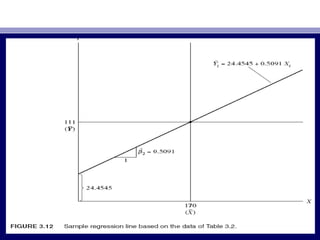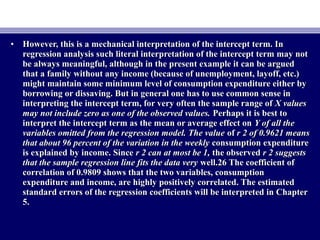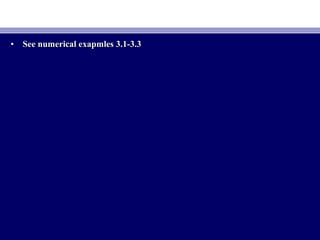1 sur 66

### Econometrics ch4

• 1. 405 ECONOMETRICS Chapter # 3: TWO-VARIABLE REGRESSION MODEL: THE PROBLEM OF ESTIMATION By Domodar N. Gujarati Prof. M. El-SakkaProf. M. El-Sakka Dept of Economics Kuwait UniversityDept of Economics Kuwait University
• 2. THE METHOD OF ORDINARY LEAST SQUARES • To understand this method, we first explain theTo understand this method, we first explain the least squares principleleast squares principle.. • Recall the two-variable PRF:Recall the two-variable PRF: YYii == ββ11 + β+ β22XXii + u+ uii (2.4.2)(2.4.2) • The PRF is not directly observable. We estimate it from the SRF:The PRF is not directly observable. We estimate it from the SRF: YYii == βˆβˆ11 + βˆ+ βˆ22XXii +uˆ+uˆii (2.6.2)(2.6.2) == YˆYˆii +uˆ+uˆii (2.6.3)(2.6.3) • wherewhere YˆYˆii is the estimated (conditional mean) value of Yis the estimated (conditional mean) value of Yii .. • But how is the SRF itself determined?But how is the SRF itself determined? First, express (2.6.3) asFirst, express (2.6.3) as uˆuˆii = Y= Yii − Yˆ− Yˆii == YYii −− βˆβˆ11 − βˆ− βˆ22XXii (3.1.1)(3.1.1) • Now givenNow given n pairs of observations on Y and X, we would like to determinen pairs of observations on Y and X, we would like to determine thethe SRFSRF in such a manner thatin such a manner that it is as close as possible to the actualit is as close as possible to the actual YY. To. To thisthis end, we may adopt the following criterion:end, we may adopt the following criterion: • Choose the SRF in such a way that the sum of the residuals ˆChoose the SRF in such a way that the sum of the residuals ˆuuii = (Y= (Yii − Yˆ− Yˆii)) isis as small as possible.as small as possible.
• 3. • But this is not a very good criterionBut this is not a very good criterion. If we adopt the criterion of. If we adopt the criterion of minimizingminimizing ˆˆuuii ,, Figure 3.1 shows that theFigure 3.1 shows that the residualsresiduals ˆˆuu22 andand ˆuˆu33 as well asas well as the residualsthe residuals ˆuˆu11 andand ˆuˆu44 receive the same weightreceive the same weight in the sumin the sum (ˆ(ˆuu11 + ˆu+ ˆu22 + ˆu+ ˆu33 + ˆu+ ˆu44)). A consequence of this is that it is quite possible that the algebraic. A consequence of this is that it is quite possible that the algebraic sum of thesum of the ˆˆuuii is small (is small (even zeroeven zero) although the) although the ˆˆuuii are widely scatteredare widely scattered about the SRF.about the SRF. • To see this, let ˆuTo see this, let ˆu11, ˆu, ˆu22, ˆu, ˆu33, and ˆu, and ˆu44 inin Figure 3.1 take the values of 10, −2,Figure 3.1 take the values of 10, −2, +2, and −10, respectively. The algebraic sum of these residuals is zero+2, and −10, respectively. The algebraic sum of these residuals is zero althoughalthough ˆˆuu11 andand ˆuˆu44 are scattered moreare scattered more widely around the SRF thanwidely around the SRF than ˆˆuu22 andand ˆuˆu33.. • We can avoid this problem if weWe can avoid this problem if we adopt theadopt the least-squares criterionleast-squares criterion, which, which states that the SRF can be fixed instates that the SRF can be fixed in such a way thatsuch a way that ˆˆuu22 ii = (Y= (Yii − Yˆ− Yˆii))22 = (= (YYii −− βˆβˆ11 − βˆ− βˆ22XXii))22 (3.1.2)(3.1.2) • is as small as possibleis as small as possible, where, where ˆuˆu22 ii are the squared residuals.are the squared residuals.
• 5. • By squaringBy squaring ˆuˆuii ,, this method gives more weight to residuals such asthis method gives more weight to residuals such as ˆˆuu11 andand ˆuˆu44 in Figure 3.1in Figure 3.1 than the residualsthan the residuals ˆuˆu22 andand ˆuˆu33.. • A further justificationA further justification for the least-squares method lies in the factfor the least-squares method lies in the fact that thethat the estimators obtained by it have some very desirable statisticalestimators obtained by it have some very desirable statistical propertiesproperties, as we shall see shortly, as we shall see shortly..
• 6. • It is obvious from (3.1.2) that:It is obvious from (3.1.2) that: ˆˆuu22 ii == f (βˆf (βˆ11, βˆ, βˆ22)) (3.1.3)(3.1.3) • that is, the sum of the squared residuals is some function of the estimatorsthat is, the sum of the squared residuals is some function of the estimators βˆβˆ11 and βˆand βˆ22. To see this. To see this, consider Table 3.1 and conduct two experiments., consider Table 3.1 and conduct two experiments.
• 7. • Since theSince the βˆβˆ values in thevalues in the two experiments are different, we gettwo experiments are different, we get different values for the estimated residualsdifferent values for the estimated residuals.. • Now which sets ofNow which sets of βˆβˆ values should we choose? Obviously thevalues should we choose? Obviously the βˆ’sβˆ’s ofof thethe first experiment are the “best” values. But we can make endlessfirst experiment are the “best” values. But we can make endless experiments and then choosing that set ofexperiments and then choosing that set of βˆβˆ values that gives us thevalues that gives us the least possible value ofleast possible value of ˆˆuu22 ii • But since time, and patience, areBut since time, and patience, are generally in short supply, we need togenerally in short supply, we need to consider some shortcuts to this trial-and-error process.consider some shortcuts to this trial-and-error process. FortunatelyFortunately,, the method of least squares provides us with unique estimates ofthe method of least squares provides us with unique estimates of ββ11 andand ββ22 thatthat give the smallest possiblegive the smallest possible value of ˆvalue of ˆuu22 ii..
• 8. ˆˆuu22 ii = (= (YYii −− βˆβˆ11 − βˆ− βˆ22XXii))22 (3.1.2)(3.1.2)
• 9. • The process of differentiation yields the following equations for estimatingThe process of differentiation yields the following equations for estimating ββ11 andand ββ22:: YYii XXii == βˆβˆ11XXii ++ βˆβˆ22XX22 ii (3.1.4)(3.1.4) YYii = n= nβˆβˆ11 + βˆ+ βˆ22XXii (3.1.5)(3.1.5) • wherewhere nn is the sample size. These simultaneous equations are known as theis the sample size. These simultaneous equations are known as the normal equationsnormal equations. Solving the normal equations simultaneously, we obtain:. Solving the normal equations simultaneously, we obtain:
• 10. • wherewhere X¯ and Y¯ are the sample means of X and Y and where weX¯ and Y¯ are the sample means of X and Y and where we definedefine xxii = (X= (Xii − X¯ )− X¯ ) andand yyii = (Y= (Yii − Y¯)− Y¯).. Henceforth we adopt theHenceforth we adopt the convention of letting theconvention of letting the lowercase letters denote deviations from meanlowercase letters denote deviations from mean valuesvalues..
• 11. • The last step in (3.1.7) can be obtained directly from (3.1.4) by simpleThe last step in (3.1.7) can be obtained directly from (3.1.4) by simple algebraic manipulations. Incidentally, note that, by making use of simplealgebraic manipulations. Incidentally, note that, by making use of simple algebraic identities, formula (3.1.6) for estimatingalgebraic identities, formula (3.1.6) for estimating ββ22 can be alternativelycan be alternatively expressed as:expressed as: • The estimators obtained previously are known as theThe estimators obtained previously are known as the least-squaresleast-squares estimatorsestimators..
• 12. • Note the followingNote the following numerical properties of estimatorsnumerical properties of estimators obtained by theobtained by the method of OLS:method of OLS: • I. The OLS estimators are expressed solely in terms of the observable (i.e.,I. The OLS estimators are expressed solely in terms of the observable (i.e., sample) quantities (i.e.,sample) quantities (i.e., X and Y).X and Y). Therefore, they can be easily computedTherefore, they can be easily computed.. • II.II. They are point estimatorsThey are point estimators; that is, given the sample, each estimator will; that is, given the sample, each estimator will provide only a single (provide only a single (point,point, not intervalnot interval) value of the relevant population) value of the relevant population parameter.parameter. • III. Once the OLS estimates are obtained from the sample data, the sampleIII. Once the OLS estimates are obtained from the sample data, the sample regression line (Figure 3.1) can be easily obtainedregression line (Figure 3.1) can be easily obtained.. • The regression lineThe regression line thus obtained has the followingthus obtained has the following propertiesproperties:: – 1. It passes through the sample means1. It passes through the sample means ofof Y and X. This fact is obviousY and X. This fact is obvious from (3.1.7), for the latter can be written asfrom (3.1.7), for the latter can be written as Y¯ = βˆY¯ = βˆ11 + βˆ+ βˆ22X¯X¯ , which is, which is shown diagrammatically in Figure 3.2.shown diagrammatically in Figure 3.2.
• 14. – 2. The mean value of the estimated2. The mean value of the estimated Y = YˆY = Yˆii is equal to the mean value ofis equal to the mean value of the actualthe actual YY for:for: YˆYˆii == βˆβˆ11 + βˆ+ βˆ22XXii = (= (Y¯ − βˆY¯ − βˆ22X¯ ) + βˆX¯ ) + βˆ22XiXi == Y¯ +Y¯ + βˆβˆ22((XXii − X¯)− X¯) (3.1.9)(3.1.9) • Summing both sides of this last equality over the sample values andSumming both sides of this last equality over the sample values and dividing through by the sample sizedividing through by the sample size nn givesgives Y¯ˆ = Y¯Y¯ˆ = Y¯ (3.1.10)(3.1.10) • where use is made of the fact thatwhere use is made of the fact that ((XXii − X¯ ) = 0− X¯ ) = 0.. – 3. The mean value of the residuals3. The mean value of the residuals ¯¯ ˆˆuuii is zerois zero.. From Appendix 3A,From Appendix 3A, Section 3A.1, the first equation is:Section 3A.1, the first equation is: −−2(2(YYii −− βˆβˆ11 − βˆ− βˆ22XXii) = 0) = 0 • But sinceBut since uˆuˆii = Y= Yii − βˆ− βˆ11 − βˆ− βˆ22XXii , the preceding equation reduces to, the preceding equation reduces to −−2 ˆ2 ˆuuii = 0, whence ¯ˆu = 0= 0, whence ¯ˆu = 0
• 15. • As a result of the preceding property, the sample regressionAs a result of the preceding property, the sample regression YYii == βˆβˆ11 + βˆ+ βˆ22XXii +uˆ+uˆii (2.6.2)(2.6.2) • can be expressed in an alternative form where bothcan be expressed in an alternative form where both Y and X are expressedY and X are expressed asas deviationsdeviations from their mean values. To see this, sum (2.6.2) on both sides tofrom their mean values. To see this, sum (2.6.2) on both sides to give:give: YYii = n= nβˆβˆ11 + βˆ+ βˆ22XXii +uˆ+uˆii == nnβˆβˆ11 + βˆ+ βˆ22XXii since uˆsince uˆii = 0= 0 (3.1.11)(3.1.11) • Dividing Eq. (3.1.11) through byDividing Eq. (3.1.11) through by n, we obtainn, we obtain Y¯ = βˆY¯ = βˆ11 + βˆ+ βˆ22X¯X¯ (3.1.12)(3.1.12) • which is the same as (3.1.7). Subtracting Eq. (3.1.12) from (2.6.2), we obtainwhich is the same as (3.1.7). Subtracting Eq. (3.1.12) from (2.6.2), we obtain YYii − Y¯ = βˆ− Y¯ = βˆ22(X(Xii − X¯ ) + uˆ− X¯ ) + uˆii • OrOr yyii == βˆβˆ22xxii +uˆ+uˆii (3.1.13)(3.1.13)
• 16. • Equation (3.1.13) is known as theEquation (3.1.13) is known as the deviation formdeviation form. Notice that the. Notice that the intercept termintercept term βˆβˆ11 is no longer present in it. But the intercept termis no longer present in it. But the intercept term cancan always be estimated by (3.1.7), that is, from the fact that the samplealways be estimated by (3.1.7), that is, from the fact that the sample regression line passes through the sample means ofregression line passes through the sample means of Y and X.Y and X. • An advantage of the deviation form is thatAn advantage of the deviation form is that it often simplifiesit often simplifies computingcomputing formulas. In passing, note that in the deviation form, theformulas. In passing, note that in the deviation form, the SRF can be written as:SRF can be written as: yˆyˆii == βˆβˆ22xxii (3.1.14)(3.1.14) • whereas in the original units of measurement it waswhereas in the original units of measurement it was YˆYˆii = βˆ= βˆ11 + βˆ+ βˆ22XXii ,, as shown in (2.6.1).as shown in (2.6.1).
• 17. – 4. The residuals ˆ4. The residuals ˆuuii are uncorrelated with the predicted Yare uncorrelated with the predicted Yii . This statement. This statement can be verified as follows: using the deviation form, we can write:can be verified as follows: using the deviation form, we can write: – where use is made of the fact thatwhere use is made of the fact that – 5.5. The residuals ˆThe residuals ˆuuii are uncorrelated with Xare uncorrelated with Xii ; that is, This; that is, This fact follows from Eq. (2) in Appendix 3A, Section 3A.1.fact follows from Eq. (2) in Appendix 3A, Section 3A.1.
• 18. THE CLASSICAL LINEAR REGRESSION MODEL: THE ASSUMPTIONS UNDERLYING THE METHOD OF LEAST SQUARES • In regression analysis our objective is not only to obtainIn regression analysis our objective is not only to obtain βˆβˆ11 andand βˆβˆ22 but also to drawbut also to draw inferencesinferences about the trueabout the true ββ11 andand ββ22. For example, we. For example, we would like to knowwould like to know howhow closeclose βˆβˆ11 and βˆand βˆ22 are to their counterparts in theare to their counterparts in the population or how closepopulation or how close YˆYˆii isis to the trueto the true E(Y | XE(Y | Xii).). • Look at the PRF:Look at the PRF: YYii = β= β11 + β+ β22XXii + u+ uii . It shows that Y. It shows that Yii depends ondepends on bothboth XXii and uand uii . The assumptions. The assumptions made about themade about the XXii variable(s) and the errorvariable(s) and the error term areterm are extremely criticalextremely critical to theto the valid interpretation of the regressionvalid interpretation of the regression estimates.estimates. • The Gaussian, standard, orThe Gaussian, standard, or classical linear regression modelclassical linear regression model ((CLRMCLRM),), makes 10 assumptionsmakes 10 assumptions..
• 19. • Keep in mind that the regressandKeep in mind that the regressand Y andY and the regressorthe regressor X themselves may beX themselves may be nonlinear.nonlinear.
• 20. • look at Table 2.1. Keeping the value of incomelook at Table 2.1. Keeping the value of income X fixed, say, atX fixed, say, at $80, we$80, we draw at random a family and observe its weekly family consumptiondraw at random a family and observe its weekly family consumption expenditureexpenditure Y as, say, $60. Still keeping X at$80, we draw at randomY as, say, $60. Still keeping X at$80, we draw at random another family and observe itsanother family and observe its Y value as $75. In each of theseY value as$75. In each of these drawingsdrawings (i.e., repeated sampling), the value of(i.e., repeated sampling), the value of X is fixed at $80. WeX is fixed at$80. We can repeat thiscan repeat this process for all theprocess for all the X values shown in Table 2.1.X values shown in Table 2.1. • This means that our regression analysis isThis means that our regression analysis is conditional regressionconditional regression analysisanalysis, that is, conditional on the given values of the regressor(s), that is, conditional on the given values of the regressor(s) X.X.
• 22. • As shownAs shown in Figure 3.3in Figure 3.3, each, each Y population corresponding to a given XY population corresponding to a given X is distributed around its mean value with someis distributed around its mean value with some Y values above theY values above the mean and some below it.mean and some below it. the mean value of these deviationsthe mean value of these deviations corresponding to any givencorresponding to any given X should be zero.X should be zero. • Note that the assumptionNote that the assumption E(uE(uii | X| Xii) = 0) = 0 implies thatimplies that E(YE(Yii | X| Xii) = β) = β11 + β+ β22XXii..
• 23. E(ui | Xi) = 0
• 24. • Technically,Technically, (3.2.2) represents the assumption of(3.2.2) represents the assumption of homoscedasticityhomoscedasticity, or, or equalequal (homo) spread (scedasticity) or equal variance. Stated differently, (3.2.2)(homo) spread (scedasticity) or equal variance. Stated differently, (3.2.2) means that themeans that the Y populations corresponding to various X values have theY populations corresponding to various X values have the same variance.same variance. • Put simply,Put simply, the variation around the regression line (which is the line ofthe variation around the regression line (which is the line of average relationship betweenaverage relationship between Y and X) is theY and X) is the samesame across the Xacross the X valuesvalues; it; it neither increases or decreases asneither increases or decreases as X variesX varies
• 27. • In Figure 3.5, where the conditional variance of theIn Figure 3.5, where the conditional variance of the YY populationpopulation varies withvaries with X. This situation is known asX. This situation is known as heteroscedasticityheteroscedasticity,, oror unequalunequal spread, or variance. Symbolically, in this situationspread, or variance. Symbolically, in this situation (3.2.2) can be(3.2.2) can be written aswritten as • var (uvar (uii | X| Xii) =) = σσ22 ii (3.2.3)(3.2.3) • Figure 3.5. shows that, var (Figure 3.5. shows that, var (u| Xu| X11) < var (u| X) < var (u| X22), . . . , < var (u| X), . . . , < var (u| Xii).). Therefore, theTherefore, the likelihood is that thelikelihood is that the Y observations coming from theY observations coming from the population with X = Xpopulation with X = X11 would be closer to the PRF than those comingwould be closer to the PRF than those coming from populations correspondingfrom populations corresponding toto X = XX = X22, X = X, X = X33, and so on. In short,, and so on. In short, notnot all Yall Y values correspondingvalues corresponding to the variousto the various X’sX’s will be equallywill be equally reliable, reliabilityreliable, reliability being judged bybeing judged by how closely or distantly thehow closely or distantly the YY values are distributed around their means, thatvalues are distributed around their means, that is, the points on theis, the points on the PRF.PRF.
• 28. • The disturbancesThe disturbances uuii and uand ujj are uncorrelated, i.e.,are uncorrelated, i.e., no serial correlation. Thisno serial correlation. This means that, givenmeans that, given XXii ,, the deviations of any two Y valuesthe deviations of any two Y values from their meanfrom their mean value do not exhibit patternsvalue do not exhibit patterns.. In Figure 3.6a, the u’s are positively correlated,In Figure 3.6a, the u’s are positively correlated, a positivea positive u followed by a positive u or a negative u followed by au followed by a positive u or a negative u followed by a negativenegative u.u. In Figure 3.6b, the u’s are negatively correlated, a positive uIn Figure 3.6b, the u’s are negatively correlated, a positive u followed by afollowed by a negativenegative u and vice versa.u and vice versa. If the disturbances follow systematic patterns,If the disturbances follow systematic patterns, Figure 3.6Figure 3.6a and b, there is auto- or serial correlation.a and b, there is auto- or serial correlation. Figure 3.6Figure 3.6c showsc shows thatthat there is no systematic pattern to thethere is no systematic pattern to the u’s, thus indicating zero correlation.u’s, thus indicating zero correlation.
• 30. • Suppose in our PRF (Suppose in our PRF (YYtt = β= β11 + β+ β22XXtt + u+ utt) that u) that utt and uand ut−1t−1 are positivelyare positively correlated.correlated. ThenThen YYtt depends not only on Xdepends not only on Xtt but also onbut also on uut−1t−1 for ufor ut−1t−1 toto some extentsome extent determinesdetermines uutt..
• 31. • The disturbanceThe disturbance u and explanatory variable Xu and explanatory variable X areare uncorrelateduncorrelated. The PRF. The PRF assumes thatassumes that XX andand uu (which may represent(which may represent the influence of all the omittedthe influence of all the omitted variables) have separate (and additive) influence onvariables) have separate (and additive) influence on YY. But if X and u are. But if X and u are correlatedcorrelated, it is not possible to assess their, it is not possible to assess their individual effects onindividual effects on Y. Thus, if XY. Thus, if X and u are positively correlated, X increasesand u are positively correlated, X increases whenwhen u increases and it decreasesu increases and it decreases when u decreases. Similarly, if X and uwhen u decreases. Similarly, if X and u are negatively correlated,are negatively correlated, X increasesX increases when u decreases and it decreaseswhen u decreases and it decreases whenwhen u increases. In either case, it isu increases. In either case, it is difficult to isolate the influence of Xdifficult to isolate the influence of X andand u on Y.u on Y.
• 32. • In the hypothetical example of Table 3.1, imagine that we had only the firstIn the hypothetical example of Table 3.1, imagine that we had only the first pair of observations onpair of observations on Y and X (4 and 1). From this single observation thereY and X (4 and 1). From this single observation there is no way to estimateis no way to estimate the two unknowns,the two unknowns, ββ11 and βand β22. We need at least two pairs. We need at least two pairs of observationsof observations to estimate the two unknownsto estimate the two unknowns
• 33. • This assumption too is not so innocuous as it looks. Look at Eq. (3.1.6). IfThis assumption too is not so innocuous as it looks. Look at Eq. (3.1.6). If all theall the X values are identical, then XX values are identical, then Xii = X¯ and the denominator of= X¯ and the denominator of thatthat equation will be zero, making it impossible to estimateequation will be zero, making it impossible to estimate β2 and therefore β1.β2 and therefore β1. Looking atLooking at our family consumption expenditure example in Chapter 2, ifour family consumption expenditure example in Chapter 2, if there is very little variation in family income, we will not be able to explainthere is very little variation in family income, we will not be able to explain much of the variation in the consumption expenditure.much of the variation in the consumption expenditure.
• 34. • An econometric investigation begins with the specification of theAn econometric investigation begins with the specification of the econometric model underlying the phenomenon of interest. Some importanteconometric model underlying the phenomenon of interest. Some important questionsquestions that arise in the specification of the model include the following:that arise in the specification of the model include the following: (1) What variables should be included in the model?(1) What variables should be included in the model? • (2) What is the functional form of the model? Is it linear in the parameters,(2) What is the functional form of the model? Is it linear in the parameters, the variables, or both?the variables, or both? • (3) What are the probabilistic assumptions made about the(3) What are the probabilistic assumptions made about the YYii , the X, the Xii, and, and the uthe uii entering the model?entering the model?
• 35. • Suppose we choose the following two models to depict the underlyingSuppose we choose the following two models to depict the underlying relationship between the rate of change of money wages and therelationship between the rate of change of money wages and the unemployment rate:unemployment rate: • YYii == αα11 + α+ α22XXii + u+ uii (3.2.7)(3.2.7) • YYii == ββ11 + β+ β22 ((11/X/Xii ) + u) + uii (3.2.8)(3.2.8) • wherewhere YYii = the rate of change of money wages, and X= the rate of change of money wages, and Xii = the unemployment= the unemployment rate. The regression model (3.2.7) is linear both in the parameters and therate. The regression model (3.2.7) is linear both in the parameters and the variables, whereas (3.2.8) is linear in the parameters (hence a linearvariables, whereas (3.2.8) is linear in the parameters (hence a linear regression model by our definition) but nonlinear in the variableregression model by our definition) but nonlinear in the variable X. NowX. Now considerconsider Figure 3.7.Figure 3.7. • If model (3.2.8) is the “correct” or the “true” model, fitting the modelIf model (3.2.8) is the “correct” or the “true” model, fitting the model (3.2.7) to the scatterpoints shown in Figure 3.7 will give us wrong(3.2.7) to the scatterpoints shown in Figure 3.7 will give us wrong predictions.predictions. • Unfortunately, in practice one rarely knows the correct variables to includeUnfortunately, in practice one rarely knows the correct variables to include in the model or the correct functional form of the model or the correctin the model or the correct functional form of the model or the correct probabilistic assumptions about the variables entering the model for theprobabilistic assumptions about the variables entering the model for the theory underlying the particular investigation may not be strong or robusttheory underlying the particular investigation may not be strong or robust
• 37. • We will discuss this assumption in Chapter 7, where we discuss multipleWe will discuss this assumption in Chapter 7, where we discuss multiple regression models.regression models.
• 38. PRECISION OR STANDARD ERRORS OF LEAST- SQUARES ESTIMATES • The least-squares estimatesThe least-squares estimates are a function of the sample dataare a function of the sample data. But since the. But since the data change from sample to sample, the estimates will change. Therefore,data change from sample to sample, the estimates will change. Therefore, what is needed is some measure of “what is needed is some measure of “reliabilityreliability” or precision of the” or precision of the estimatorsestimators βˆβˆ11 andand βˆβˆ22. In statistics the precision of an estimate is measured by. In statistics the precision of an estimate is measured by itsits standardstandard error (se),error (se), which can be obtained as follows:which can be obtained as follows:
• 39. • σσ22 is the constantis the constant oror homoscedastichomoscedastic variance ofvariance of uuii of Assumption 4.of Assumption 4. • σσ22 itself isitself is estimated by the following formula:estimated by the following formula: • wherewhere ˆˆσσ22 is the OLS estimator of the trueis the OLS estimator of the true but unknown σbut unknown σ22 and where theand where the expressionexpression n−2 is known as the number of degrees of freedom (df), isn−2 is known as the number of degrees of freedom (df), is the residual sum of squares (the residual sum of squares (RSSRSS). Once). Once is known, ˆis known, ˆσσ22 can be easilycan be easily computed.computed. • Compared with Eq. (3.1.2), Eq. (3.3.6) is easy to use, for it does not requireCompared with Eq. (3.1.2), Eq. (3.3.6) is easy to use, for it does not require computing ˆcomputing ˆuuii for each observationfor each observation..
• 40. • SinceSince • anan alternative expressionalternative expression for computing isfor computing is • In passing, note that the positive square root of ˆIn passing, note that the positive square root of ˆσσ22 • is known as theis known as the standard error of estimatestandard error of estimate or the standard error of theor the standard error of the regression (se). It is simply the standard deviation of theregression (se). It is simply the standard deviation of the Y values aboutY values about thethe estimated regression line and isestimated regression line and is often used as a summary measure of theoften used as a summary measure of the “goodness of fit“goodness of fit” of the estimated regression line.” of the estimated regression line.
• 41. • Note theNote the following features of the variancesfollowing features of the variances (and therefore the standard(and therefore the standard errors) oferrors) of βˆβˆ11 andand βˆβˆ22.. • 1. The variance of1. The variance of βˆβˆ22 is directly proportional tois directly proportional to σσ22 but inversely proportionalbut inversely proportional toto xx22 ii . That is, given. That is, given σσ22 , the larger the variation in the, the larger the variation in the XX values, thevalues, the smaller thesmaller the variance ofvariance of βˆβˆ22 and hence the greater the precision with whichand hence the greater the precision with which ββ22 can becan be estimated.estimated. • 2. The variance of2. The variance of βˆβˆ11 is directly proportional tois directly proportional to σσ22 andand XX22 ii but inverselybut inversely proportional toproportional to xx22 ii and the sample sizeand the sample size nn..
• 42. • 3. Since3. Since βˆβˆ11 and βˆand βˆ22 are estimators, they will not only vary from sample toare estimators, they will not only vary from sample to samplesample but in a given sample theybut in a given sample they are likely to be dependent onare likely to be dependent on each other,each other, this dependence being measured by the covariance between them.this dependence being measured by the covariance between them. • Since var (Since var (βˆβˆ22) is) is alwaysalways positivepositive, as is the variance of any variable, the, as is the variance of any variable, the naturenature of the covarianceof the covariance betweenbetween βˆβˆ11 andand βˆβˆ22 depends on the sign of X¯ . If X¯ isdepends on the sign of X¯ . If X¯ is positive,positive, then as the formula shows, the covariance will bethen as the formula shows, the covariance will be negativenegative. Thus, if. Thus, if the slope coefficientthe slope coefficient ββ22 is overestimated (i.e., the slope is too steep), theis overestimated (i.e., the slope is too steep), the interceptintercept coefficientcoefficient ββ11 will be underestimated (i.e., the intercept will be toowill be underestimated (i.e., the intercept will be too small).small).
• 43. PROPERTIES OF LEAST-SQUARES ESTIMATORS: THE GAUSS–MARKOV THEOREM • To understand this theorem, we need to consider the best linearTo understand this theorem, we need to consider the best linear unbiasedness property of an estimator. An estimator, say the OLS estimatorunbiasedness property of an estimator. An estimator, say the OLS estimator βˆβˆ22, is said to be a best linear unbiased, is said to be a best linear unbiased estimatorestimator (BLUE) of(BLUE) of ββ22 if the followingif the following hold:hold: • 1.1. It is linearIt is linear, that is, a linear function of a random variable, such as the, that is, a linear function of a random variable, such as the dependent variabledependent variable Y in the regression model.Y in the regression model. • 2.2. It is unbiasedIt is unbiased, that is, its, that is, its averageaverage or expected value,or expected value, E(βˆE(βˆ22), is equal to), is equal to thethe true value,true value, ββ22.. • 3.3. It has minimum varianceIt has minimum variance in the class of all such linear unbiasedin the class of all such linear unbiased estimators; an unbiased estimator with the least variance is known as anestimators; an unbiased estimator with the least variance is known as an efficient estimator.efficient estimator.
• 44. • What all this means can be explained with the aid of Figure 3.8. In FigureWhat all this means can be explained with the aid of Figure 3.8. In Figure 3.8(3.8(a) we have shown the sampling distribution of the OLSa) we have shown the sampling distribution of the OLS estimatorestimator βˆβˆ22, that, that is, the distribution of the values taken by βˆis, the distribution of the values taken by βˆ22 in repeatedin repeated sampling experiment.sampling experiment. For convenience we have assumedFor convenience we have assumed βˆβˆ22 to be distributed symmetricallyto be distributed symmetrically. As the. As the figure shows, the mean of thefigure shows, the mean of the βˆβˆ22 values, E(βˆvalues, E(βˆ22), is equal to the true β), is equal to the true β22. In this. In this situation we say thatsituation we say that βˆβˆ22 is an unbiased estimator of βis an unbiased estimator of β22. In Figure 3.8(b) we. In Figure 3.8(b) we have shown the sampling distribution ofhave shown the sampling distribution of β∗β∗22, an alternative estimator of β, an alternative estimator of β22 obtained by using another (i.e., other than OLS) method.obtained by using another (i.e., other than OLS) method.
• 46. • For convenience, assume thatFor convenience, assume that β*β*22, like, like βˆβˆ22, is unbiased, that is, its average or, is unbiased, that is, its average or expected value isexpected value is equal toequal to ββ22. Assume further that both βˆ. Assume further that both βˆ22 and β*and β*22 are linearare linear estimators, that is, they are linear functions ofestimators, that is, they are linear functions of Y. Which estimator, βˆY. Which estimator, βˆ22 or β*or β*22,, would you choose? To answer this question, superimpose the two figures, aswould you choose? To answer this question, superimpose the two figures, as in Figure 3.8(in Figure 3.8(c).c). It is obvious that although bothIt is obvious that although both βˆβˆ22 and β*and β*2 are unbiased2 are unbiased the distribution ofthe distribution of β*β*22 is more diffused or widespread around the meanis more diffused or widespread around the mean value than the distribution ofvalue than the distribution of βˆβˆ22. In other words, the variance of β*. In other words, the variance of β*22 is largeris larger than the variance ofthan the variance of βˆβˆ22.. • Now given two estimators that are both linear and unbiased, one wouldNow given two estimators that are both linear and unbiased, one would choose the estimator with the smaller variance because it is more likely tochoose the estimator with the smaller variance because it is more likely to be close tobe close to ββ22 than the alternative estimator.than the alternative estimator. In short, one would choose theIn short, one would choose the BLUE estimator.BLUE estimator.
• 47. THE COEFFICIENT OF DETERMINATION r2 : A MEASURE OF “GOODNESS OF FIT” • We now consider theWe now consider the goodness of fitgoodness of fit of the fitted regression line to a set ofof the fitted regression line to a set of data; that is, we shall find out how “well” the sample regression line fits thedata; that is, we shall find out how “well” the sample regression line fits the data. The coefficient of determinationdata. The coefficient of determination rr22 (two-variable case) or(two-variable case) or RR22 (multiple(multiple regression) is a summaryregression) is a summary measure that tells howmeasure that tells how well the sample regressionwell the sample regression line fits the dataline fits the data.. • Consider a heuristic explanationConsider a heuristic explanation ofof rr22 in terms of a graphical device, knownin terms of a graphical device, known as the Venn diagramas the Venn diagram shown in Figure 3.9.shown in Figure 3.9. • In this figure the circleIn this figure the circle Y represents variation in the dependent variable YY represents variation in the dependent variable Y andand the circlethe circle X represents variation in the explanatory variable X. TheX represents variation in the explanatory variable X. The overlap ofoverlap of the two circles indicates the extent to which the variation inthe two circles indicates the extent to which the variation in Y is explainedY is explained by the variation in X.by the variation in X.
• 49. • To compute thisTo compute this rr22 , we proceed as follows: Recall that, we proceed as follows: Recall that • YYii = Yˆ= Yˆii +uˆ+uˆii (2.6.3)(2.6.3) • or in the deviation formor in the deviation form • yyii = ˆy= ˆyii + ˆu+ ˆuii (3.5.1)(3.5.1) • where use is made of (3.1.13) and (3.1.14). Squaring (3.5.1) on both sideswhere use is made of (3.1.13) and (3.1.14). Squaring (3.5.1) on both sides and summing over the sample, we obtainand summing over the sample, we obtain • • SinceSince = 0 and yˆ= 0 and yˆii = βˆ= βˆ22xxii ..
• 62. • βˆβˆ11 = 24.4545= 24.4545 var (var (βˆβˆ11) = 41.1370) = 41.1370 andand se (se (βˆβˆ11) = 6.4138) = 6.4138 • βˆβˆ22 = 0.5091= 0.5091 var (var (βˆβˆ22) = 0.0013) = 0.0013 andand se (se (βˆβˆ22) = 0.0357) = 0.0357 • cov (cov (βˆβˆ11, βˆ, βˆ22) = −0.2172) = −0.2172 σˆσˆ22 = 42.1591= 42.1591 (3.6.1)(3.6.1) • rr22 = 0.9621= 0.9621 r = 0.9809r = 0.9809 df = 8df = 8 • The estimated regression line therefore isThe estimated regression line therefore is • YˆYˆii = 24.4545 + 0.5091X= 24.4545 + 0.5091Xii (3.6.2)(3.6.2) • which is shown geometrically as Figure 3.12.which is shown geometrically as Figure 3.12. • Following Chapter 2, the SRF [Eq. (3.6.2)] and the associated regressionFollowing Chapter 2, the SRF [Eq. (3.6.2)] and the associated regression line are interpreted as follows: Each point on the regression line gives anline are interpreted as follows: Each point on the regression line gives an estimate of the expected or mean value of Y corresponding to the chosen Xestimate of the expected or mean value of Y corresponding to the chosen X value; that is,value; that is, YˆYˆii is an estimate of E(Y | Xis an estimate of E(Y | Xii). The value of βˆ). The value of βˆ22 = 0.5091, which= 0.5091, which measures the slope of the line, shows that, within the sample range ofmeasures the slope of the line, shows that, within the sample range of XX between $80 and$260 per week, asbetween $80 and$260 per week, as X increases, say, by $1, the estimatedX increases, say, by$1, the estimated increase in the mean or average weekly consumption expenditure amountsincrease in the mean or average weekly consumption expenditure amounts to about 51 cents. The value ofto about 51 cents. The value of βˆβˆ11 = 24.4545, which is the intercept of the= 24.4545, which is the intercept of the line, indicates the average level of weekly consumption expenditure whenline, indicates the average level of weekly consumption expenditure when weekly income is zero.weekly income is zero.